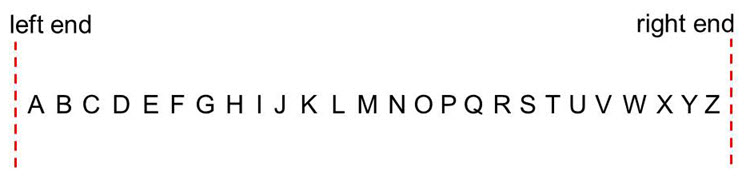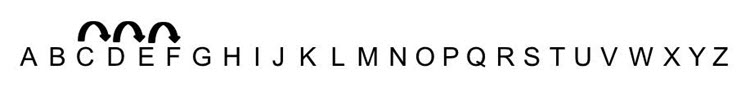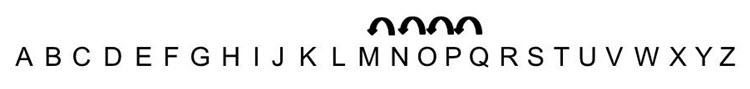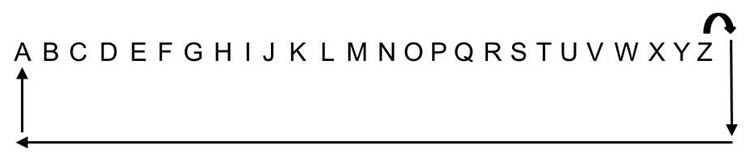## How To Solve Type 1 Problems

#### Step 1 - Check to see if it is a “mirror code” problem

We define a “mirror” as being a letter of the alphabet that is equidistant from another letter of the alphabet about the midpoint of the alphabet. For example, in the diagram below the midpoint of the alphabet is between the letters M and N and the letter M is the “mirror” of the letter N because M is one to the left of the midpoint and N is one to the right of the midpoint. Similarly, J is the “mirror” of Q, since they are both 4 from the midpoint and S is the “mirror” of H since they are both 6 from the midpoint.You can also think of “mirrors” as being letters that are equidistant from the left end of the alphabet and the right end of the alphabet shown in the diagram below. For example, from the diagram below you can see that B is the “mirror” of Y since B is the second letter from the left end of the alphabet and Y is the 2nd letter from the right end of the alphabet. Similarly, D is the “mirror” of W since they are both 4 from the ends of the alphabet, and X is the “mirror” of C since they are both 3 from the ends of the alphabet.The first thing you should do when solving type 1 problems is to see if you are dealing with “mirrors” and whether the solution is a “mirror code”.

For example, suppose you have the problem

If the code for CAT is XZG,
what is the code for CARD?

This is a “mirror code” problem because the letters are “mirrors” i.e. C is the “mirror” of X, A is the “mirror” of Z and T is the “mirror” of G. Therefore the answer is the “mirror code” of CARD which is XZIW, because X is the “mirror” of C, Z is the “mirror” of A, I is the “mirror” of R and W is the “mirror” of D.

#### Step 2 - If it is not a “mirror code” problem, use a forward backward movement approach

If the letters are not “mirrors” and it is not a “mirror code” problem, then the problem can be solved by using a forward backward movement approach.

We treat a forward movement as being a movement to the right and as being a positive movement (i.e. a + movement). For example, C to F is +3 (i.e. a forward movement of 3 to the right) as shown in the diagram belowWe treat a backward movement as being a movement to the left and as being a negative movement (i.e. a – movement). For example, Q to M is -4 (i.e. a backward movement of 4 to the left) as shown in the diagram belowNote that a forward (rightward) movement of 1 (a movement of +1) from the end of the alphabet, Z, goes to the start of the alphabet, A, as shown in the diagram belowand a backward (leftward) movement of 1 (a movement of -1) from the start of the alphabet, A, goes to the end of the alphabet, Z, as shown in the diagram belowThere are two types of problem that can be solved using the forward backward movement approach i.e. “what is the code for a word?” and “what does the code mean?”

What is the code for a word?

Suppose you have the problem

If the code for BAY is EFD,
what is the code for HOT?

This is not a “mirror code” problem because the letters are not “mirrors”. Therefore, use a forward backward movement approach.

B to E is +3 (i.e. a forward, rightward movement of 3)
A to F is +5 (i.e. a forward, rightward movement of 5)
Y to D is +5 (i.e. a forward, rightward movement of 5)

So to go from the word BAY to the code EFD we need to apply the movement +3 +5 +5 to the word BAY

To find the code for the word HOT (i.e. to go from the word HOT to its code) we need to apply these same movements of +3, +5 and + 5 to the letters H, O and T and doing this we get

H +3 is K
O +5 is T
T +5 is Y

so the code for HOT is KTY

What does the code mean?

Suppose you have the problem

If the code for ANT is YIP,
what does DPJ mean?

This is not a “mirror code” problem because the letters are not “mirrors”. Therefore, use a forward backward movement approach.

A to Y is -2 (i.e. a backward, leftward movement of 2)
N to I is -5 (i.e. a backward, leftward movement of 5)
T to P is -4 (i.e. a backward, leftward movement of 4)

The question is “what does DPJ mean?”. Therefore DPJ is a code and we have to find the meaning of this code i.e. we have to find the word which has the code DPJ.

We are told that the code for ANT is YIP i.e.
to go from the word ANT to the code YIP is a movement of -2 -5 -4
and therefore to go the opposite way, from the code YIP to the word ANT is a movement in the opposite direction i.e. is a movement of +2 +5 +4

Therefore to go from the code DPJ to the word that it stands for, we need to apply +2 +5 +4 to the code DPJ which gives us the word FUN because

D +2 is F
P +5 is U
J +4 is N